| home      | people     | research     | publications    | seminars    | events     | contactCommunication Networks   | Systems Biology   | Hybrid Systems    | Machine Learning   | Dynamics & Interaction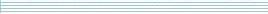### Schools Mathematics Grand Challenge

Week eight's Puzzles

Problem 22:

The twenty second problem was:

John has been given a field in the shape of a triangle. Two sides of the triangle are exactly 10 metres long. What is the largest possible area, in square metres, of John's triangular field? Give your answer as the number of square metres. There is no need to include units.

The solution was:

The area of a triangle is half the base by the perpendicular height. We get to choose which side to use as the base, so let's use one of the sides of John's field that is length 10 metres.Since the base is now fixed, we get the biggest area by making the height be as big as possible. The height cannot be bigger than 10, otherwise the second side of length 10 would not be able to reach the corner, so we get the biggest area by putting the two sides at right angles. The area will then be 10*10/2 = 50.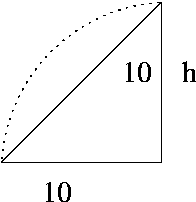Problem 23:

The twenty third problem was:

Have a look at the following picture. The circle is area 438 units and has centre C. The line from A to E goes through the centre and the line from D to B goes through the centre. The angle between CA and AB is 60 degrees.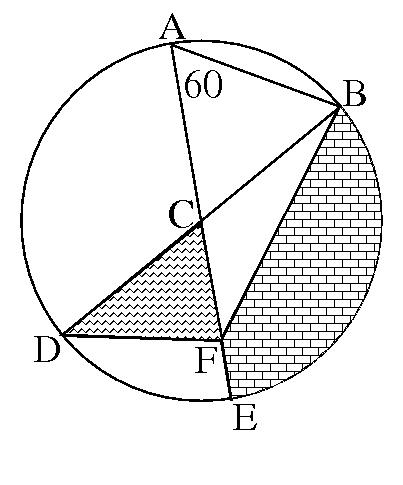If the area of the zig-zaggy triangle CDF is 30 units, what is the area of the bricked piece of the picture? Again, there is no need to give units.

The solution was:

We want to find the area of the bricked bit of the picture. It is the area of the segment of the circle cut out by the line BC, the line CE and the arc EB minus the area of the triange BCF. Let's figure out the area of both these parts.

We've been told that the angle CAB is 60, but CA and CB are both radii of the circle, so ACB is isosceles and the angle CBA must be 60 degrees too. Since the angle in a triangle add up to 180, ACB must be 60. Finally, since ACE is a straight line, the angle BCE must be 120. The angles are shown on the picture below.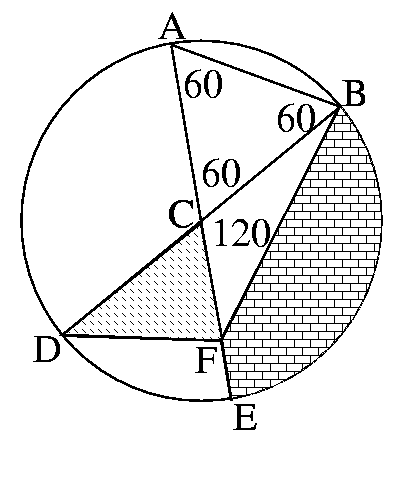Now 120 degrees is one third of the circle. We were told that the full area of the circle was 438, so the area of the segment BCE must be 438/3 = 146.

Now we need to figure out the area of the triangle BCF. Imagine that CB is the base and the heigth of point F is the height. CB is a radius of the circle, so it the same as CD. The point F is also the same height above DC ad CB, so BCF and CDF have the same base length and the same heigth. This means they must have the same area, which we was told was 30 units.

So, the area of the bricked bit of the picture is

(area of the segment BCE) - (area of the triangle BCF) = 438/3 - 30 = 116.

Problem 24:

The twenty fourth problem was:

In the triangle in the diagram below, the length of the side AB is the same as the length of the side AC. The point N divides the line from B to C into two equal parts. Also, the line BM divides the angle ABC into two equal parts, and the line from B to M is two times as long as the line from A to N.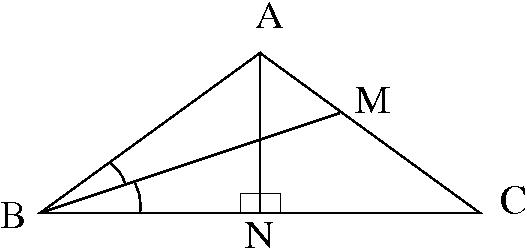What is the size, in degrees, of the angle BAC (the largest angle) in the triangle?

The solution was:

We're going to draw an extra line to make the problem a bit easier. Let's draw a line through N parallel to BM, like this.The triangle NCP will be a scaled down version of BCM, because all the angles are the same and the BC is twice as long as NC. This means that BM is twice the length of NP, but we were told that AN was half the length of BM, so AN and NP must be the same length. So ANP is an isosceles triangle.

Now, let's mark in some angles, shown on the picture below. Let the two half-angles at the corned B be a. Then, since ABC is isosceles, the angle at C must be 2a also. Since MBC and PNC are similar, the angle at PNC must also be a. Call the angle at BAN b, then NAC is also b and because ANP is an isosceles triangle NPA must also be b. The last angle we need to mark is the one at NPC, call this c.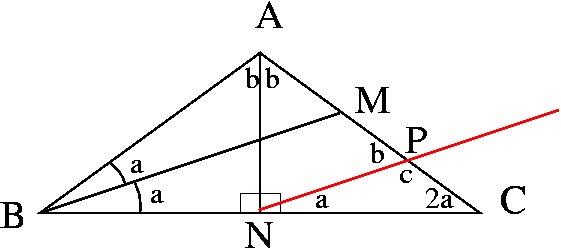Now APC is a straight line we get c = 180 - b. Now, look at triangle NPC, we know the angles add up to 180, so a + c + 2a = 180, or 3a + 180 - b = 180, so 3a = b. Finally, we look at the triangle ABC we get 2a + 2b + 2a = 180, or 4a+2b = 180, or 4a + 6a = 180, so a = 18, b = 54 and the angle at the top top of the triangle is 2b = 108.

Phew!